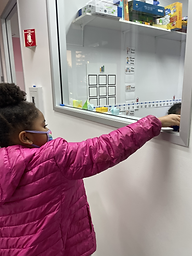Ms. Caitlyn

Target 1​

Lesson Type:

New

Algebra

:

Classification

Classify objects based on some property they have in common.

1:

Use a classification key.

2:

In a given group of objects, determine which objects does not fit with the others.

3:

Name the property that objects have in common.

1st

Vocabulary:

Classification, Category

Activities:

• Students classified animals by their habitats, then created a classification key.
• Students classified shapes by color and size.
• Students classified 2D and 3D shapes, then created a classification key.Home Exploration

Guiding Questions:Absent Students:

Target 2

:

1:

Understand that a graph is a visual representation of data.

2:

Become familiar with the vocabulary associated with graphs (title and labels).

3:

Construct a simple bar graph.

2nd

Vocabulary:

Bar Graph, Title, Label, Category

Activities:

• Students went on a scavenger hunt for six types of fruit, then sorted the fruit into categories.
• Students counted the fruit and organized them into a bar graph using blocks.
• Students picked four handfuls of fruit and sorted them into categories, then graphed them using graph paper.Home Exploration

Guiding Questions:Target 3

:

1:

Solve problems that have more than one operation, addition and subtraction.

2:

Understand when solving a problem that has both addition and subtraction operations that solving starts on the left and continues to the right.

2nd

Vocabulary:

Tens, Ones

Activities:

• Students rolled dice to generate numbers in order to create a multi-step problem.
• Students solved multi-step problems using base ten blocks.Home Exploration